# Chapter 4 Mass and Energy Analysis of Control

• Slides: 36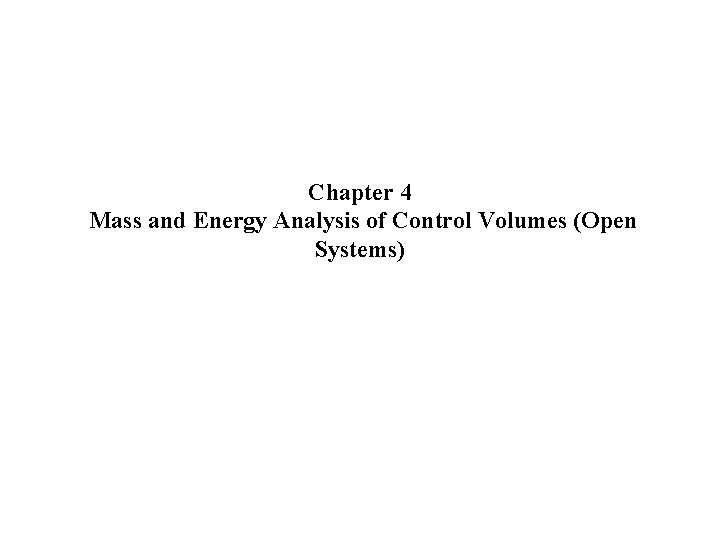Chapter 4 Mass and Energy Analysis of Control Volumes (Open Systems)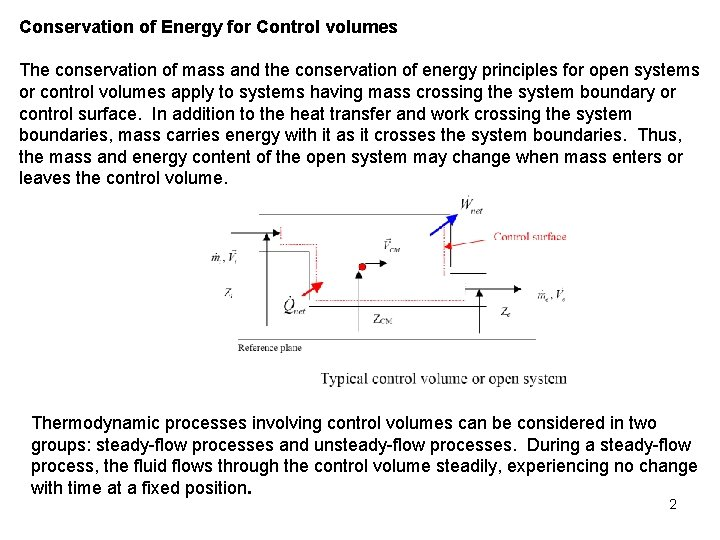Conservation of Energy for Control volumes The conservation of mass and the conservation of energy principles for open systems or control volumes apply to systems having mass crossing the system boundary or control surface. In addition to the heat transfer and work crossing the system boundaries, mass carries energy with it as it crosses the system boundaries. Thus, the mass and energy content of the open system may change when mass enters or leaves the control volume. Thermodynamic processes involving control volumes can be considered in two groups: steady-flow processes and unsteady-flow processes. During a steady-flow process, the fluid flows through the control volume steadily, experiencing no change with time at a fixed position. 2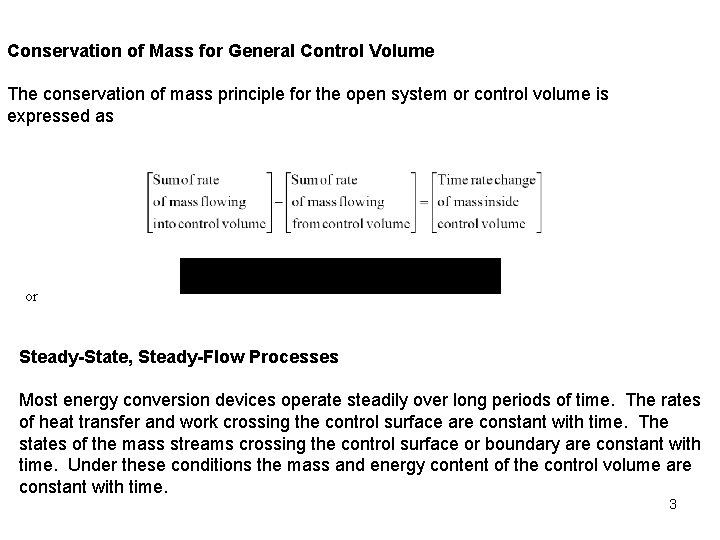Conservation of Mass for General Control Volume The conservation of mass principle for the open system or control volume is expressed as or Steady-State, Steady-Flow Processes Most energy conversion devices operate steadily over long periods of time. The rates of heat transfer and work crossing the control surface are constant with time. The states of the mass streams crossing the control surface or boundary are constant with time. Under these conditions the mass and energy content of the control volume are constant with time. 3Steady-state, Steady-Flow Conservation of Mass: Since the mass of the control volume is constant with time during the steady-state, steady-flow process, the conservation of mass principle becomes or Special Case: Steady Flow of an Incompressible Fluid The mass flow rate is related to volume flow rate and fluid density by For one entrance, one exit steady flow control volume, the mass flow rates are related by 4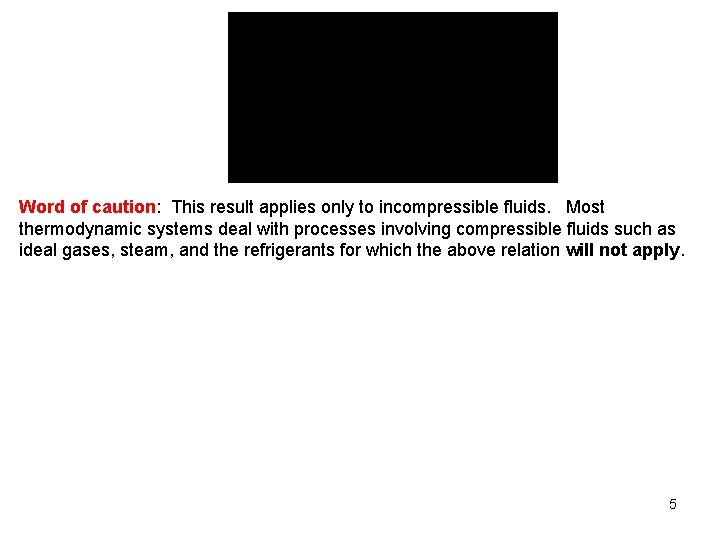Word of caution: This result applies only to incompressible fluids. Most thermodynamic systems deal with processes involving compressible fluids such as ideal gases, steam, and the refrigerants for which the above relation will not apply. 5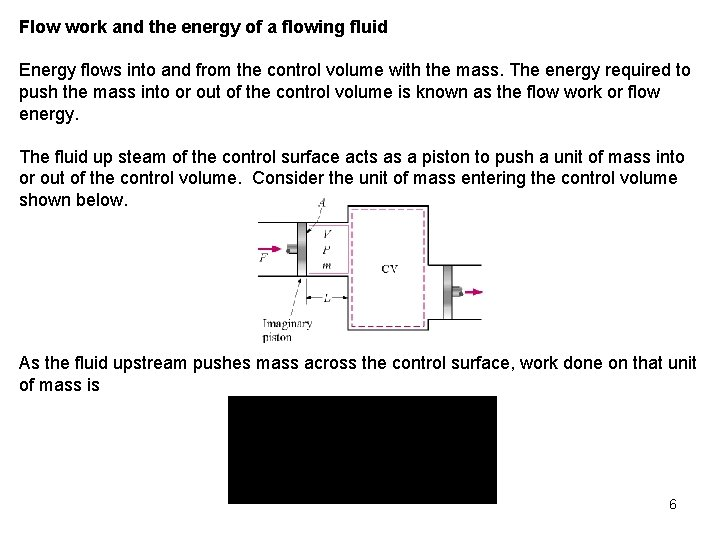Flow work and the energy of a flowing fluid Energy flows into and from the control volume with the mass. The energy required to push the mass into or out of the control volume is known as the flow work or flow energy. The fluid up steam of the control surface acts as a piston to push a unit of mass into or out of the control volume. Consider the unit of mass entering the control volume shown below. As the fluid upstream pushes mass across the control surface, work done on that unit of mass is 6The term Pv is called the flow work done on the unit of mass as it crosses the control surface. The total energy of flowing fluid The total energy carried by a unit of mass as it crosses the control surface is the sum of the internal energy, flow work, potential energy, and kinetic energy. Here we have used the definition of enthalpy, h = u + Pv. Energy transport by mass Amount of energy transport across a control surface: 7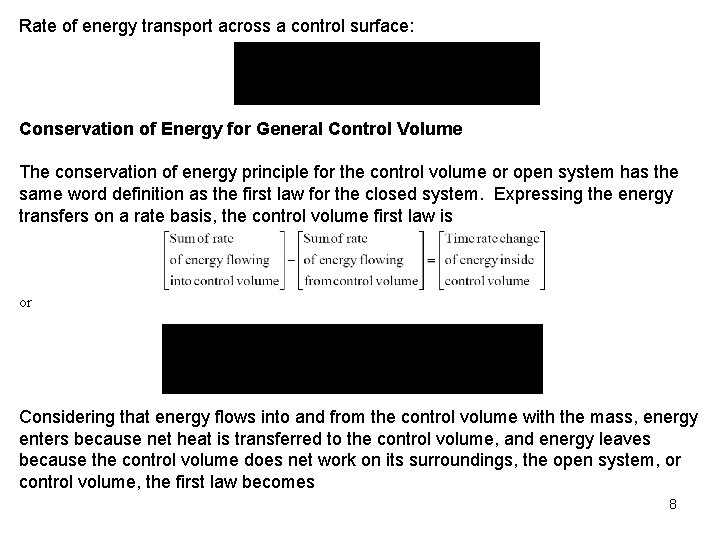Rate of energy transport across a control surface: Conservation of Energy for General Control Volume The conservation of energy principle for the control volume or open system has the same word definition as the first law for the closed system. Expressing the energy transfers on a rate basis, the control volume first law is or Considering that energy flows into and from the control volume with the mass, energy enters because net heat is transferred to the control volume, and energy leaves because the control volume does net work on its surroundings, the open system, or control volume, the first law becomes 8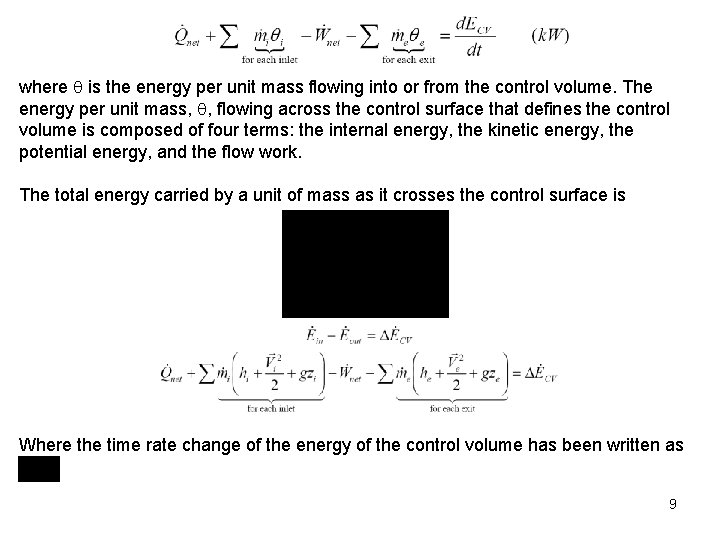where is the energy per unit mass flowing into or from the control volume. The energy per unit mass, , flowing across the control surface that defines the control volume is composed of four terms: the internal energy, the kinetic energy, the potential energy, and the flow work. The total energy carried by a unit of mass as it crosses the control surface is Where the time rate change of the energy of the control volume has been written as 9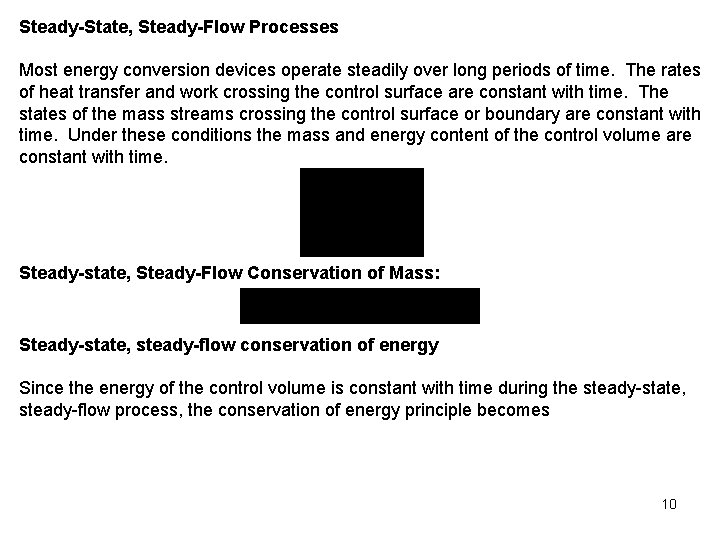Steady-State, Steady-Flow Processes Most energy conversion devices operate steadily over long periods of time. The rates of heat transfer and work crossing the control surface are constant with time. The states of the mass streams crossing the control surface or boundary are constant with time. Under these conditions the mass and energy content of the control volume are constant with time. Steady-state, Steady-Flow Conservation of Mass: Steady-state, steady-flow conservation of energy Since the energy of the control volume is constant with time during the steady-state, steady-flow process, the conservation of energy principle becomes 10or 0 or Considering that energy flows into and from the control volume with the mass, energy enters because heat is transferred to the control volume, and energy leaves because the control volume does work on its surroundings, the steady-state, steady-flow first law becomes 11Often this result is written as where Steady-state, steady-flow for one entrance and one exit A number of thermodynamic devices such as pumps, fans, compressors, turbines, nozzles, diffusers, and heaters operate with one entrance and one exit. The steadystate, steady-flow conservation of mass and first law of thermodynamics for these systems reduce to 12where the entrance to the control volume is state 1 and the exit is state 2 and mass flow rate through the device. is the When can we neglect the kinetic and potential energy terms in the first law? Consider the kinetic and potential energies per unit mass. 13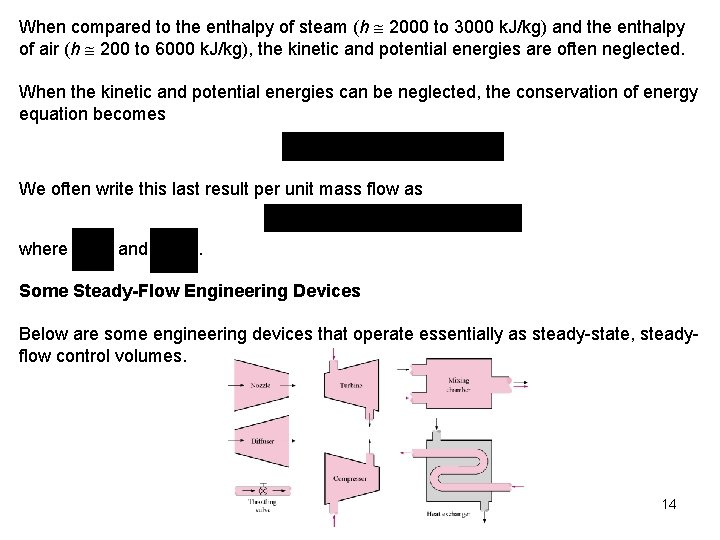When compared to the enthalpy of steam (h 2000 to 3000 k. J/kg) and the enthalpy of air (h 200 to 6000 k. J/kg), the kinetic and potential energies are often neglected. When the kinetic and potential energies can be neglected, the conservation of energy equation becomes We often write this last result per unit mass flow as where and . Some Steady-Flow Engineering Devices Below are some engineering devices that operate essentially as steady-state, steadyflow control volumes. 14Nozzles and Diffusers For flow through nozzles, the heat transfer, work, and potential energy are normally neglected, and nozzles have one entrance and one exit. The conservation of energy becomes 15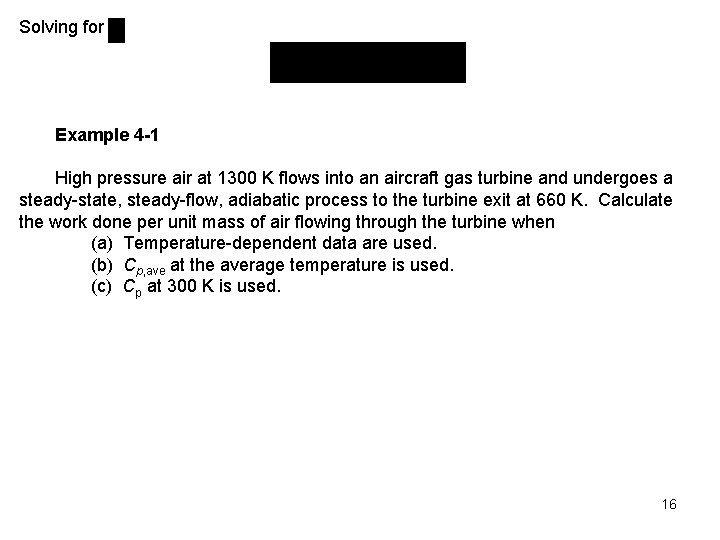Solving for Example 4 -1 High pressure air at 1300 K flows into an aircraft gas turbine and undergoes a steady-state, steady-flow, adiabatic process to the turbine exit at 660 K. Calculate the work done per unit mass of air flowing through the turbine when (a) Temperature-dependent data are used. (b) Cp, ave at the average temperature is used. (c) Cp at 300 K is used. 16Control Volume: The turbine. Property Relation: Assume air is an ideal gas and use ideal gas relations. Process: Steady-state, steady-flow, adiabatic process Conservation Principles: Conservation of mass: Conservation of energy: According to the sketched control volume, mass and work cross the control surface. Neglecting kinetic and potential energies and noting the process is adiabatic, we have 17The work done by the air per unit mass flow is Notice that the work done by a fluid flowing through a turbine is equal to the enthalpy decrease of the fluid. (a) Using the air tables, Table A-17 at T 1 = 1300 K, h 1 = 1395. 97 k. J/kg at T 2 = 660 K, h 2 = 670. 47 k. J/kg 18(b) Using Table A-2(c) at Tave = 980 K, Cp, ave = 1. 138 k. J/kg K (c) Using Table A-2(a) at T = 300 K, Cp = 1. 005 k. J/kg K Compressors and fans 19Compressors and fans are essentially the same devices. However, compressors operate over larger pressure ratios than fans. If we neglect the changes in kinetic and potential energies as fluid flows through an adiabatic compressor having one entrance and one exit, the steady-state, steady-flow first law or the conservation of energy equation becomes Example 4 -2 Nitrogen gas is compressed in a steady-state, steady-flow, adiabatic process from 0. 1 MPa, 25 o. C. During the compression process the temperature becomes 125 o. C. If the mass flow rate is 0. 2 kg/s, determine the work done on the nitrogen, in k. W. 20Control Volume: The compressor (see the compressor sketched above) Property Relation: Assume nitrogen is an ideal gas and use ideal gas relations Process: Adiabatic, steady-flow Conservation Principles: Conservation of mass: Conservation of energy: According to the sketched control volume, mass and work cross the control surface. Neglecting kinetic and potential energies and noting the process is adiabatic, we have for one entrance and one exit 21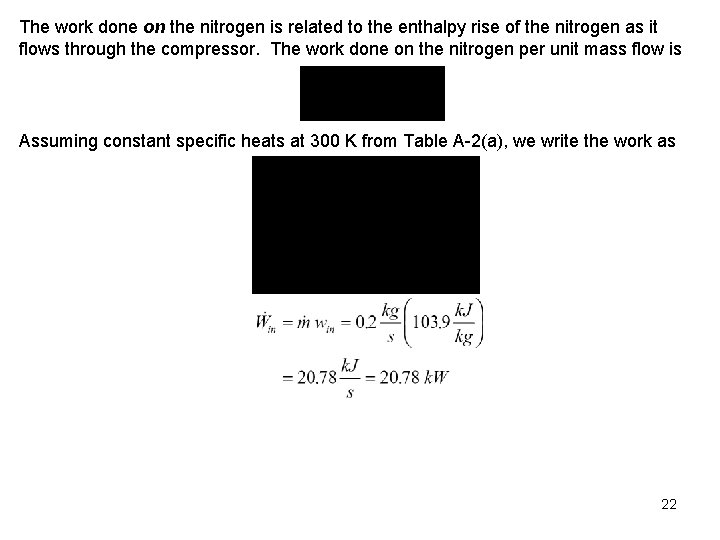The work done on the nitrogen is related to the enthalpy rise of the nitrogen as it flows through the compressor. The work done on the nitrogen per unit mass flow is Assuming constant specific heats at 300 K from Table A-2(a), we write the work as 22Throttling devices Consider fluid flowing through a one-entrance, one-exit porous plug. The fluid experiences a pressure drop as it flows through the plug. No net work is done by the fluid. Assume the process is adiabatic and that the kinetic and potential energies are neglected; then the conservation of mass and energy equations become 23This process is called a throttling process. What happens when an ideal gas is throttled? When throttling an ideal gas, the temperature does not change. We will see later in Chapter 11 that the throttling process is an important process in the refrigeration cycle. A throttling device may be used to determine the enthalpy of saturated steam. The steam is throttled from the pressure in the pipe to ambient pressure in the calorimeter. The pressure drop is sufficient to superheat the steam in the calorimeter. Thus, the temperature and pressure in the calorimeter will specify the enthalpy of the steam in the pipe. 24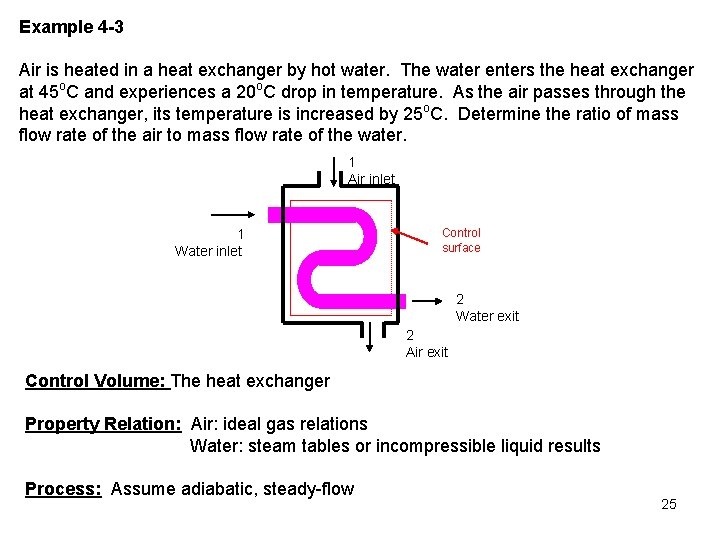Example 4 -3 Air is heated in a heat exchanger by hot water. The water enters the heat exchanger at 45 o. C and experiences a 20 o. C drop in temperature. As the air passes through the heat exchanger, its temperature is increased by 25 o. C. Determine the ratio of mass flow rate of the air to mass flow rate of the water. 1 Air inlet 1 Water inlet Control surface 2 Water exit 2 Air exit Control Volume: The heat exchanger Property Relation: Air: ideal gas relations Water: steam tables or incompressible liquid results Process: Assume adiabatic, steady-flow 25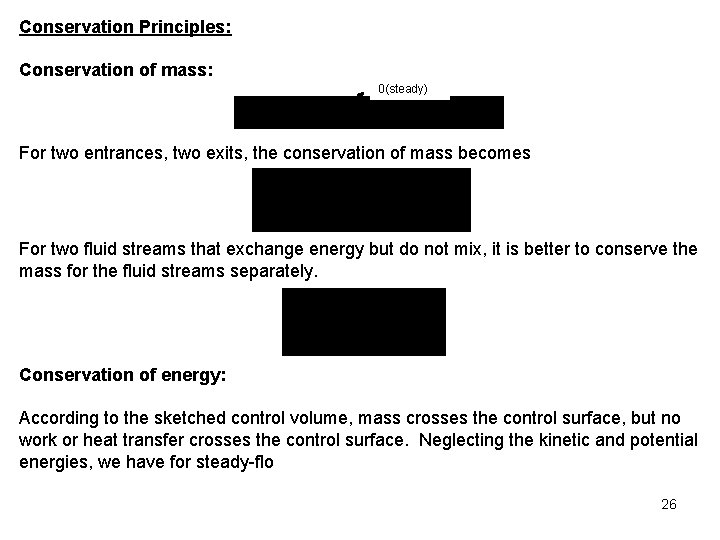Conservation Principles: Conservation of mass: 0(steady) For two entrances, two exits, the conservation of mass becomes For two fluid streams that exchange energy but do not mix, it is better to conserve the mass for the fluid streams separately. Conservation of energy: According to the sketched control volume, mass crosses the control surface, but no work or heat transfer crosses the control surface. Neglecting the kinetic and potential energies, we have for steady-flo 260(steady) We assume that the air has constant specific heats at 300 K, Table A-2(a) (we don't know the actual temperatures, just the temperature difference). Because we know the initial and final temperatures for the water, we can use either the incompressible fluid result or the steam tables for its properties. Using the incompressible fluid approach for the water, Table A-3, Cp, w = 4. 18 k. J/kg K. 27A second solution to this problem is obtained by determining the heat transfer rate from the hot water and noting that this is the heat transfer rate to the air. Considering each fluid separately for steady-flow, one entrance, and one exit, and neglecting the kinetic and potential energies, the first law, or conservation of energy, equations become 28Example 4 -4 Air at 100 o. C, 0. 15 MPa, 40 m/s, flows through a converging duct with a mass flow rate of 0. 2 kg/s. The air leaves the duct at 0. 1 MPa, 113. 6 m/s. The exit-to-inlet duct area ratio is 0. 5. Find the required rate of heat transfer to the air when no work is done by the air. 1 Air inlet Air exit 2 Control surface Control Volume: The converging duct Property Relation: Assume air is an ideal gas and use ideal gas relations Process: Steady-flow Conservation Principles: Conservation of mass: 29Conservation of energy: According to the sketched control volume, heat transfer and mass cross the control surface, but no work crosses the control surface. Here keep the kinetic energy and still neglect the potential energies, we have for steady-state, steady-flow process 0(steady) In the first law equation, the following are known: P 1, T 1 (and h 1), , and A 2/A 1. The unknowns are , and h 2 (or T 2). We use the first law and the conservation of mass equation to solve for the two unknowns. 30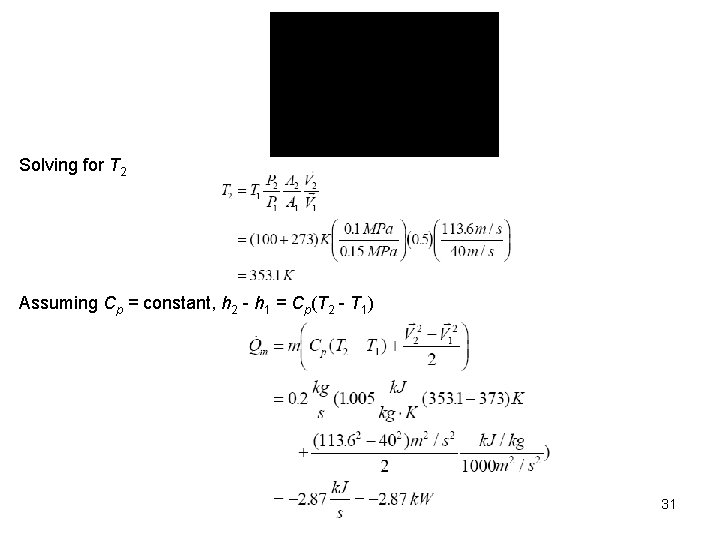Solving for T 2 Assuming Cp = constant, h 2 - h 1 = Cp(T 2 - T 1) 31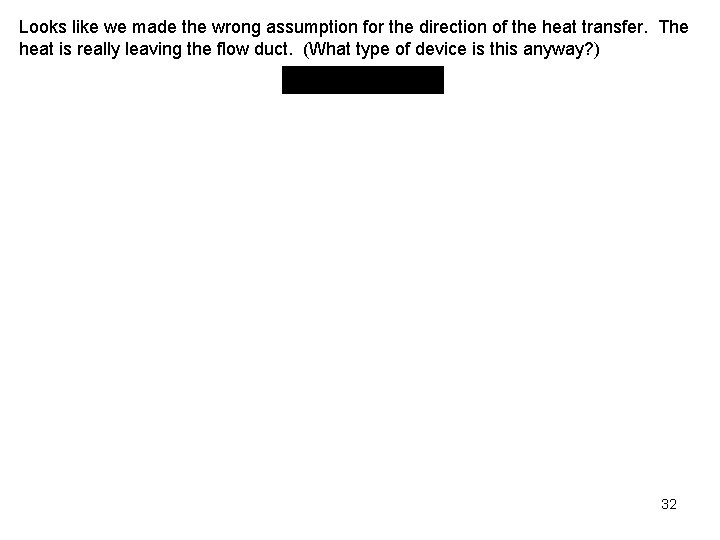Looks like we made the wrong assumption for the direction of the heat transfer. The heat is really leaving the flow duct. (What type of device is this anyway? ) 32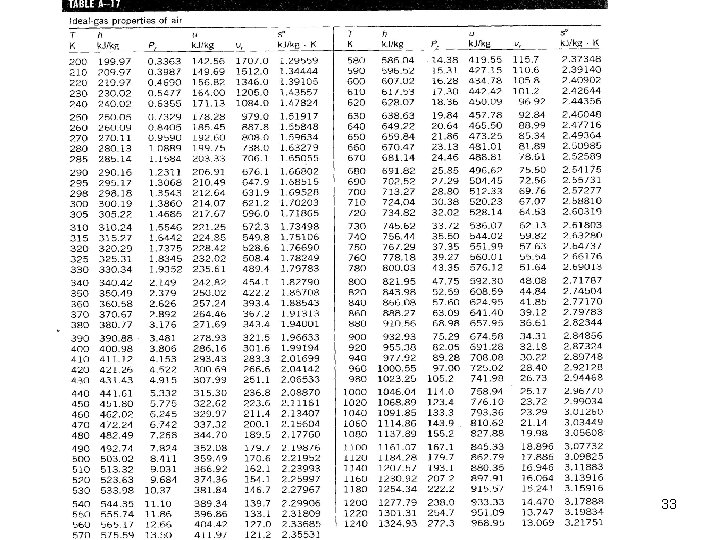33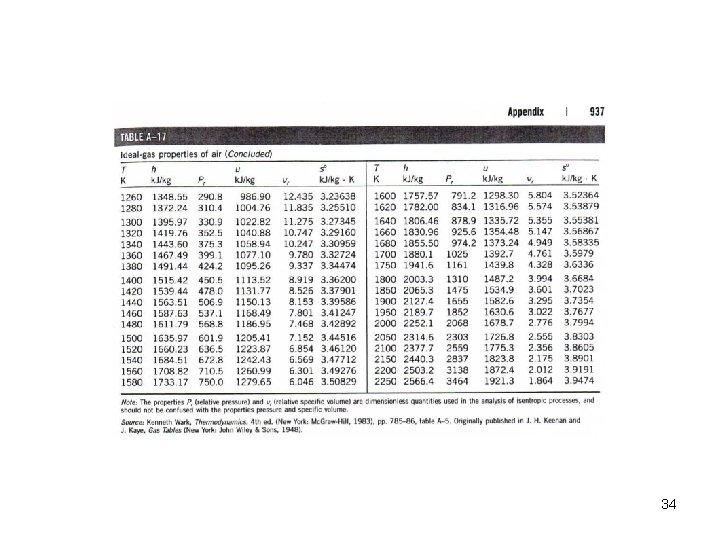343536